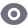# 程序验证（一）：命题逻辑

## 概念

### 命题逻辑

• 一个原子(atom)是一个或为真或为假的判断。
• 一个文字(literal)是一个原子或它的非。
• 命题公式(propositional formulas)由文字和逻辑连接符组成。

### 合式公式

• $\langle atom\rangle$ ::= $\top (true) | \bot (false)|P,Q, ... (命题变量)$
• $\langle literal\rangle$::=$\langle atom\rangle$|$\neg$$\langle atom\rangle$（非）
• $\langle formula\rangle$::=$\langle literal\rangle$
|$\neg$$\langle formula\rangle$ (negation)
|$\langle formula\rangle$ $\wedge$$\langle formula\rangle$ (conjunction)
|$\langle formula\rangle$ $\vee$$\langle formula\rangle$ (disjunction)
|$\langle formula\rangle$ $\to$$\langle formula\rangle$ (implication)
|$\langle formula\rangle$ $\leftrightarrow$$\langle formula\rangle$ (equivalence)

$\top$
$P\wedge (Q \vee R\to P)$
$P$
$P\Rightarrow Q$

### 语义

$Formula: F + Interpretation: I=TruthValue(true, false)$

$I={P\mapsto true, Q\mapsto false, R\mapsto false,...}$

$I \models F$

$I \not \models F$

$F: P\wedge Q \to P\vee \neg Q$
$I:\{P\mapsto true,Q\mapsto false\}$

Step No. Step Reason
(1) $I\models P$ $I[P]=true$
(2) $I\not\models Q$ $I[Q]=false$
(3) $I\models\neg Q$ $(2) and \neg$
(4) $I\not\models P\wedge Q$ $(2) and \wedge$
(5) $I\models P\wedge Q\to P\vee \neg Q$ $(4) and \to$

### 可满足性与永真性

• 真值表穷举：蛮力搜索复杂度为$2^n$，其中$n$为变元的数量
• 演绎推理：语义讨论
1. 假定$F$为非永真的，即存在$I$$I\not \models F$
2. 应用推理规则
3. 如果每一分支都得到矛盾，那么$F$是永真的
4. 如果没有矛盾，且不能进一步应用推理规则，那么$F$是非永真的

## 语义规则

### 公式

$\frac{I\models \neg F}{I\not \models F}$
$\frac{I\not \models \neg F}{I\models F}$
$\frac{I\models F\wedge G}{I\models F ~ I\models G}$
$\frac{I\not\models F\wedge G}{I\not \models F | I\not\models G}$这个规则产生了分支
$\frac{I\models F\vee G}{I\models F | I\models G}$
$\frac{I\not\models F\vee G}{I\not\models F~I\not \models G}$
$\frac{I\models F\to G}{I\not\models F | I\models G}$
$\frac{I\not\models F\to G}{I\models F~I\not\models G}$
$\frac{I\models F\leftrightarrow G}{I\models F\wedge G|I\models \neg F\wedge \neg G}$
$\frac{I\not \models F\leftrightarrow G}{I\models F\wedge \neg G|I\models \neg F\wedge G}$

### 举例

1. $F: P\wedge Q$

1 $I\not\models P\wedge Q$ assumption
2 $I\not\models P 1 and $\wedge$, case A 3$I\not\models Q 1 and $\wedge$, case B
1. $F: P\wedge Q \to P\vee \neg Q$

1 $I\not \models F$ assum.
2 $I\models P\wedge Q$ 1 and $\to$
3 $I\not\models P\vee\neg Q$ 1 and $\to$
4 $I\models P$ 2 and $\wedge$
5 $I\not\models P$ 3 and $\vee$
6 $\bot$ 4 and 5

## 范式(Normal Forms)

### 概念

• 限制公式的语法(Restricts the syntax of formulas)
• 对于此逻辑中的任意公式，范式具有等价的表达能力(Has equivalent representation for any formula in the logic)

### 三种主要范式

#### Conjunctive Normal Form (CNF)

$\neg P\wedge\neg(P\vee Q)$是NNF
$\neg P\wedge\neg Q\vee R$是DNF
$(\neg P\vee Q)\wedge (P\to R)$是CNF
$\neg P\wedge P$是CNF

### 范式转化

02-24355

#### 离散数学【01】——逻辑和证明

05-03249

#### 读《一本小小的蓝色逻辑书》：识别常见的逻辑漏洞02-291万+

#### 《Java语言程序设计与数据结构》编程练习答案（第九章）

04-275917

#### 离散数学：验证P,Q两个逻辑表达式是否逻辑等价(C语言实现)

02-281万+

#### 《Java语言程序设计与数据结构》编程练习答案（第七章）（一）

09-23303

#### [离散] 打印任意命题公示的真值表和主范式

04-11486

#### 联结词的逻辑运算

06-30148

#### 《程序员的数学：2逻辑》

05-031万+

#### c语言标准库详解（九）：实用函数stdlib.h

01-10764

#### 命题逻辑自动证明机（C#实现）

04-301万+

#### c语言标准库详解（八）：数学公式math.h

09-12812

#### 离散结构：基础：逻辑和证明（Discrete Structures ：The Foundations: Logic and Proofs）(3)

05-29228

#### 《python基础教程》答案（第五章）

02-24377

#### 《一本小小的蓝色逻辑书》读书笔记

04-156582

#### 命题逻辑等值演算

03-27111

#### 《CSAPP》（第3版）答案（第三章）（一）

04-262810

03-11

#### HoloLens2开发入门教程

05-01©️2020 CSDN 皮肤主题: 鲸 设计师: meimeiellie点击重新获取扫码支付1.余额是钱包充值的虚拟货币，按照1:1的比例进行支付金额的抵扣。
2.余额无法直接购买下载，可以购买VIP、C币套餐、付费专栏及课程。余额充值